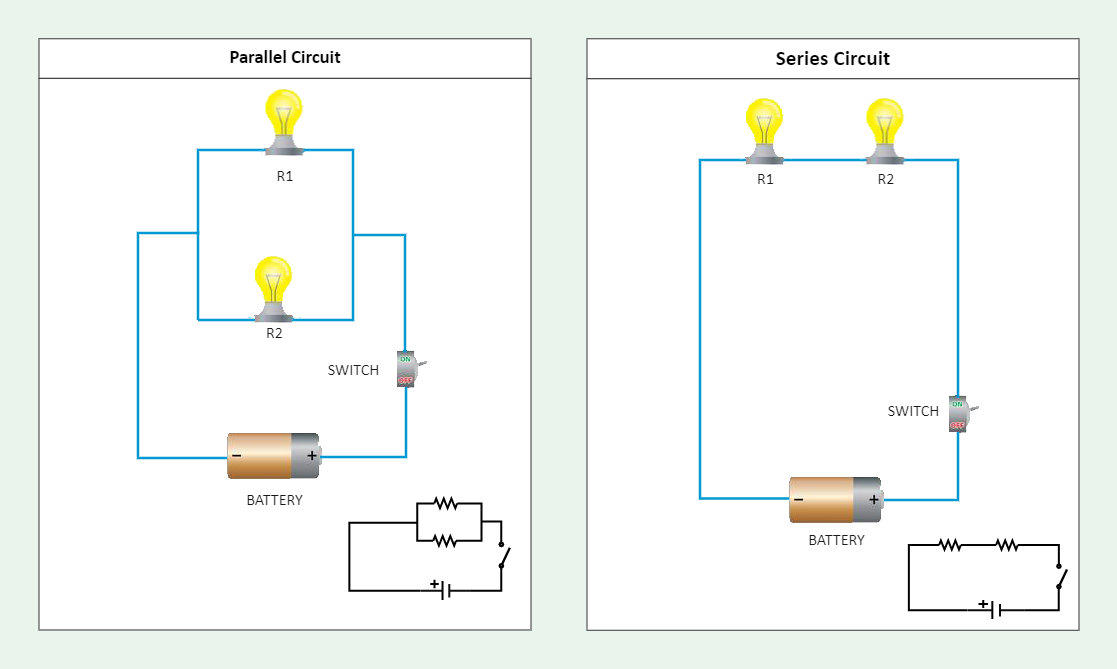Template Community / Serial Circuit Diagram

# Serial Circuit DiagramBlackwood
Published on 2022-03-23
Edit OnlineIntroduction
A circuit diagram represents how different components are connected in a device and shows the current flow pathway. There are different types of circuits, such as serial and parallel circuits. In simple terms, if the components are connected in series, it becomes a serial circuit, and if connected in parallel, it is called a parallel circuit. In the serial circuit, the current has only one path to take. The current is the same through each resistor.

As shown in the serial circuit diagram below, the amount of current flowing through the two appliances is the same in a series connection. In the case of a parallel connection, the voltage across each appliance is the same. A parallel circuit can consume more power when compared to a series circuit. Let us understand more about it through the serial circuit diagram and how easy it is to create one using EdrawMax Online.

1. Understanding Serial Circuit Diagram
Since you know what a circuit diagram is, it is easy to understand a serial circuit diagram. This simple circuit diagram, which comprises resistors, a battery, and a switch, shows the current flow. A bulb glows at the output if the circuit is connected accurately. The serial circuit is powered by an energy source, which is the battery. Two resistors are connected in series with each other.

There is only one way for the current to flow in the serial circuit diagram. Starting from the battery's positive terminal, current flow will lead to resistor 1. From there, the current will flow straight to R2 and finally back to the battery's negative terminal. Here, there is only one path for current to follow.

Nevertheless, in the case of a parallel circuit, current flows from the positive battery terminal, current flows to R1 and R2. The node that connects the battery to R1 is also connected to the other resistors. The other ends of these resistors are similarly tied together and then tied back to the battery's negative terminal. The current can take two distinct paths before returning to the battery, and the associated resistors are said to be in parallel.

2. ​​How to Create a Serial Circuit Diagram using EdrawMax Online?
Creating a Serial Circuit Diagram in EdrawMax Online is pretty simple. The free Electrical Circuit maker has several features as you can instantly import the images or icons from your system, Google Drive, or DropBox. Your Electrical Circuit diagram will require additional media content, making it more creative.

Choose a template
EdrawMax Online comes with hundreds of free diagram templates. Select a pre-designed template by entering the Keyword in the "Search" section or exploring different diagram sets. In this case, you will find different types of Electrical Circuits diagrams under the "Electrical Engineering" section under the "Engineering" section. You will find different diagram sets, like Basic Electrical, Circuits and Logic, Industrial Control System, and Systems' Diagram. Alternatively, you can simply click on "+" in EdrawMax Online canvas to create a diagram of your preference.

Upon your research, you will find that a series circuit comprises a path along which the whole current flows through each component in a serial circuit diagram. A parallel circuit comprises branches so that the current divides and only part of it flows through any branch.

Customize the diagram
Customize your Serial Circuit Diagram by changing the color or adding more relevant data. You can also add or remove the Electrical Circuit symbols or content accordingly based on your research. Since it is about electrical engineering research, you can add more relevant data about different circuit diagrams.

Export & Share
Once your required circuit diagram is completed, you can share it amongst your colleagues or clients using the easy export and share option. You can further export the diagram in multiple formats, like Graphics, JPEG, PDF, or HTML. Also, you can share the Serial Circuit Diagram designs on different social media platforms, like Facebook, Twitter, LinkedIn, or Line. In simple words, you can export your incredible Electrical Circuit diagrams to the files you want with just a few clicks.

3. Important Points to Consider While Creating Serial Circuit Diagram
While designing a circuit diagram, there are several points to be considered. A small mistake in the circuit can harm the complete system. The same is the case with a serial circuit diagram. Below mentioned are certain points that you should consider while designing the circuit.

We can use as many resistors as we need, but make sure to connect them in series with each other.
Cross-check the connections from positive and negative terminals of the battery. A small change can harm the complete circuit.
Use a two-way switch in the circuit.
You may use blocks to create the diagram or go with conventional symbols.

Conclusion
If the connections are made clear, the bulb glows in a serial and parallel circuit. Hopefully, you must have understood the functioning of each component in the circuit. Use EdrawMax Online to create a better-looking Circuit Diagram for your projects. The free electrical circuit diagram maker offers free symbols, templates, and other diagram elements that ease your effort in making the serial circuit diagram for your project.
Tag
circuit diagram
Circuit Diagram Collection
wiring plan diagram
Report
1
161PostRecommended Templates